# Insert a node at a specific position in a linked list

## Introduction

In this blog, we will discuss a linked list problem that has been asked frequently in Interviews.

The problem is to Insert a node at a specific position in a linked list.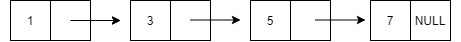Now, coming to the question.

We are given N elements in a linked list, and we are asked to insert an element at a given specified position.

For example: here at position 2, we are asked to insert 2.After inserting,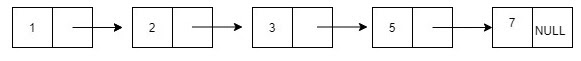## Approach

Now we will discuss the approach to Insert a node at a specific position in a linked list.

Declare the structure of the Node and write the function to create and return the node.

Now for inserting the Node, check if the required position is valid or not.

Create a new Node.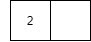1)New Node should point the old Node at the same position.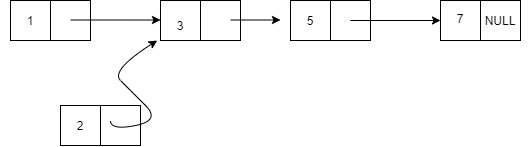2) Change the pointer of the Node previous to the old Node to point to the new Node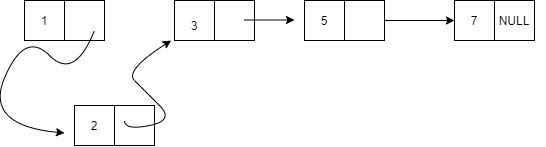Time Complexity = O(n)

Since in the worst case, only one traversal is needed.

Till now, I assume you must have got the basic idea of what has been asked in the problem statement. So, I strongly recommend you first give it a try.

And solve some related problems on the linked list.

If you could not solve how to Insert a node at a specific position in a linked list, don’t be sad. This is part of the learning process.

Please have a look at the algorithm, and then again, you must give it a try.

### PseudoCode

Algorithm

___________________________________________________________________

procedure insert( ):

___________________________________________________________________

1.   If n==1:

return

3.   Traverse till the required position and then go its link.

4.   After traversing, update the link.

temp1->next=temp2->next;

temp2->next=temp1;

end procedure

___________________________________________________________________
Let's take a quick look at a video to have a visual idea of how to insert a node at a specific position in a linked list.

## CODE IN C++

``````// Insert a node at a specific position in a linked list
#include <bits/stdc++.h>
using namespace std;

// Declaration of node of a linked list
struct Node {
int data;
struct Node* next;
};

// function to insert a Node at the mentioned position
void insert(int data, int n)
{
Node* temp1 = new Node();
temp1->data = data;
temp1->next = NULL;

// function to insert node at position 1.
if(n==1){
return;
}

//inserting at the given position

//traversing
for(int i=0; i<n-2; i++){
temp2=temp2->next;
}

temp1->next=temp2->next;
temp2->next=temp1;

}

//function to display the node
void print(){
while(temp!=NULL){
printf("%d ",temp->data);
temp=temp->next;
}
printf("\n");
}

// Driver Code
int main()
{
// Creating the list 1->3->5->7
insert(1,1);
insert(3,2);
insert(5,3);
insert(7,4);

insert(2,2);
print();
return 0;
}``````

Output

``````Input:  1 3 5 7
Output:  1 2 3 5 7``````

### Complexity Analysis

Time Complexity: O(n).

Analyzing Time Complexity:

In the worst case, all elements are to be traversed once.

∴n.

Space complexity: O(1) since no extra variable is used.

1. Can we access a node directly in a linked list?
Answer) Unlike an array, this is not possible in a linked list.

2. What things should we keep in mind for such a problem?
Answer) Ensure that you are not de-attaching all the nodes in a single time. Make sure that the nodes exist in the problem.

3. Is it necessary to initialise the last node address as null?
Answer) Yes, as elements are not stored in a contiguous manner, pointers are used, so sometimes it may take the garbage value.

4. Why do sometimes I get a segmentation fault?
Answer) This is most likely if we try to access NULL or out of bounds memory.

## Key Takeaways

This article taught us how to Insert a node at a specific position in a linked list. We discussed its implementation using illustrations, pseudocode, and then proper code.

We hope you could take away critical techniques like analysing problems by walking over the execution of the examples and finding out the recursive pattern followed in most linked list problems.

Now, we recommend you practice problem sets based on the linked list to master your fundamentals. You can get a wide range of questions similar to this on CodeStudio